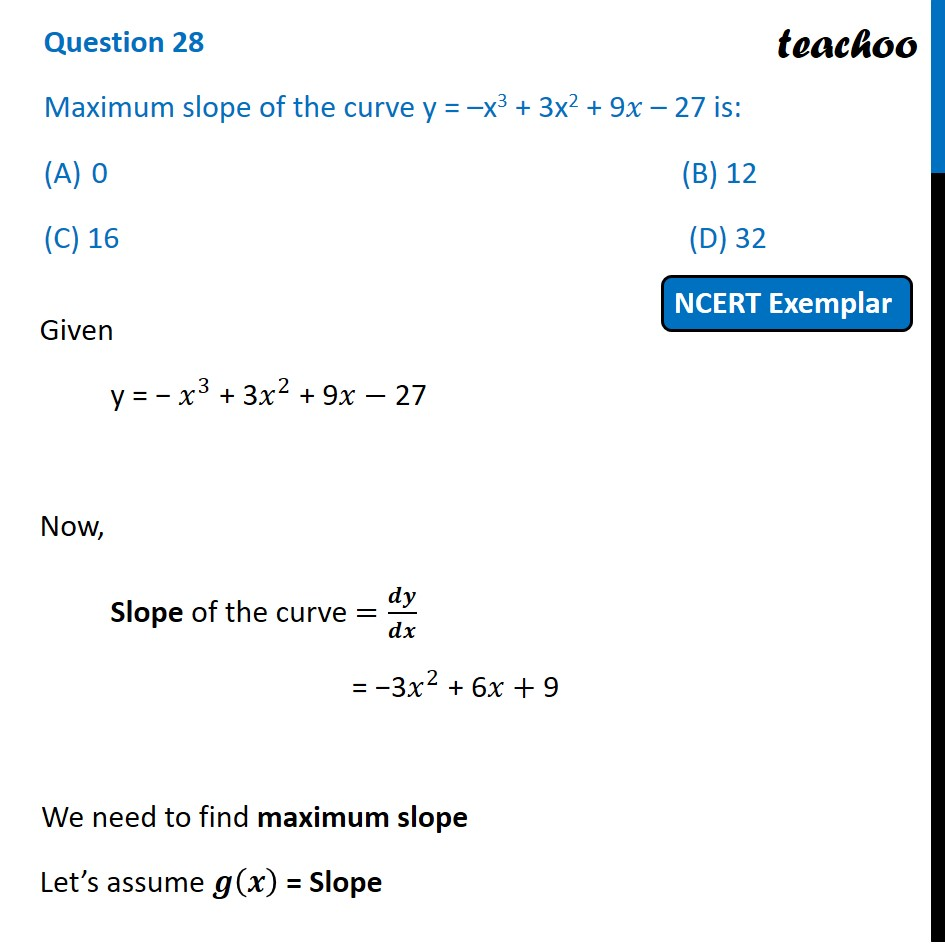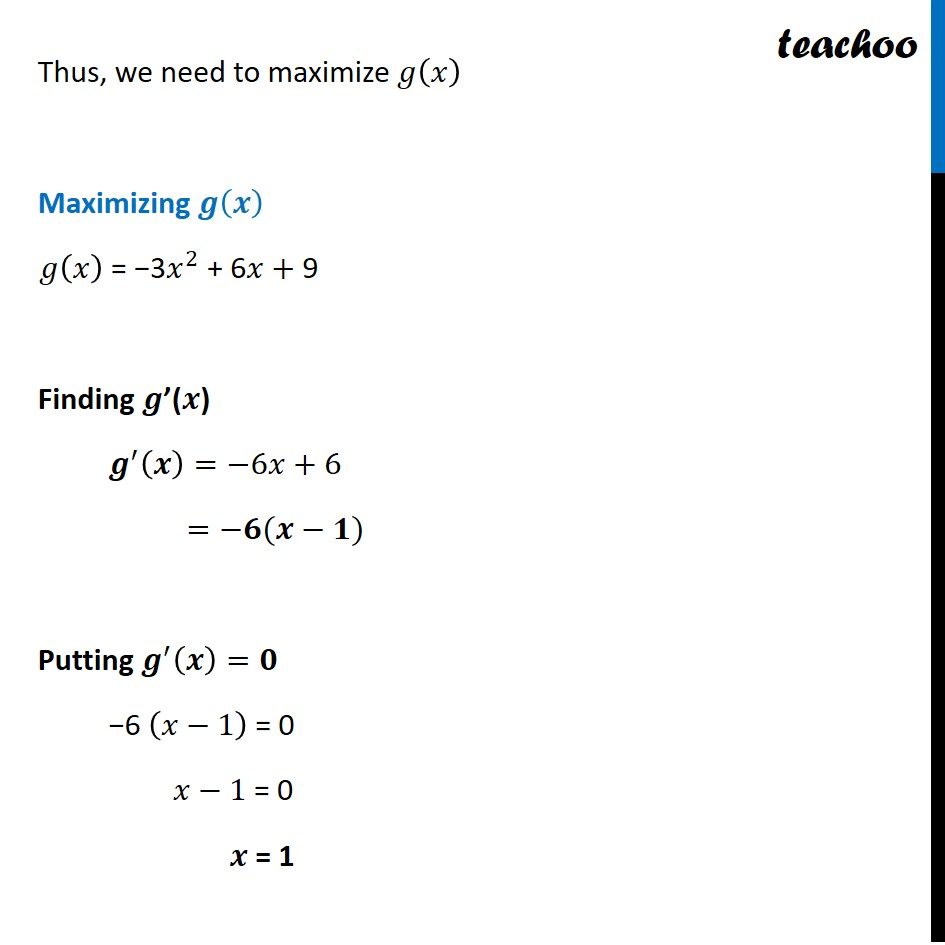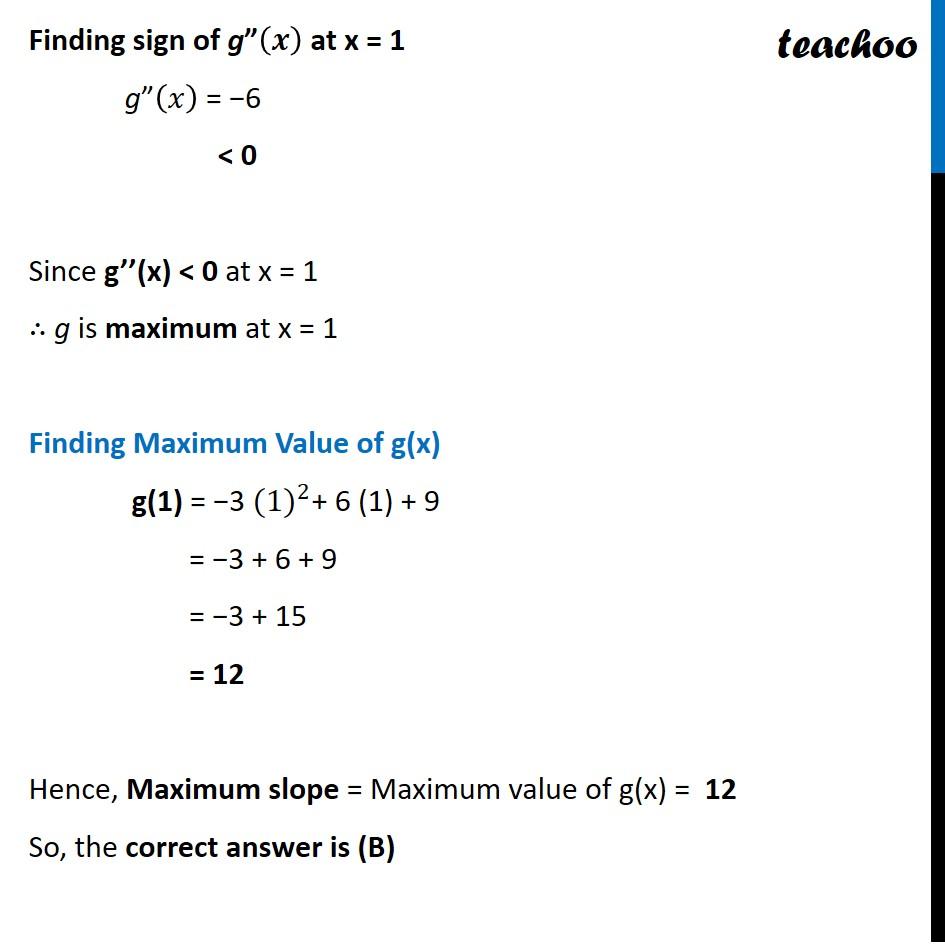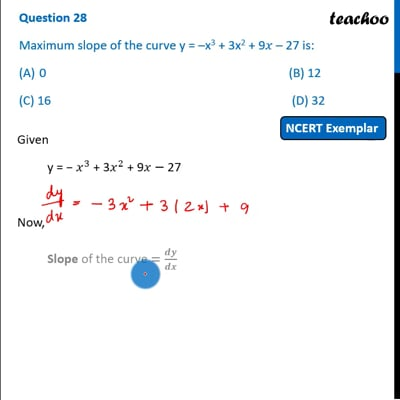NCERT Exemplar - MCQs

Chapter 6 Class 12 Application of Derivatives
Serial order wise

## (C) 16                 (D) 32This video is only available for Teachoo black users

Learn in your speed, with individual attention - Teachoo Maths 1-on-1 Class

### Transcript

Question 15 Maximum slope of the curve y = –x3 + 3x2 + 9𝑥 – 27 is: 0 (B) 12 (C) 16 (D) 32 Given y = − 𝑥^3 + 3𝑥^2 + 9𝑥− 27 Now, Slope of the curve =𝒅𝒚/𝒅𝒙 = −3𝑥^2 + 6𝑥+ 9 We need to find maximum slope Let’s assume 𝒈(𝒙) = Slope Thus, we need to maximize 𝑔(𝑥) Maximizing 𝒈(𝒙) 𝑔(𝑥) = −3𝑥^2 + 6𝑥+ 9 Finding 𝒈’(𝒙) 𝒈^′ (𝒙)=−6𝑥+6 =−𝟔(𝒙−𝟏) Putting 𝒈^′ (𝒙)=𝟎 −6 (𝑥−1) = 0 𝑥−1 = 0 𝒙 = 1 Finding sign of g”(𝒙) at x = 1 g”(𝑥) = −6 < 0 Since g’’(x) < 0 at x = 1 ∴ g is maximum at x = 1 Finding Maximum Value of g(x) g(1) = "−3 " 〖(1)〗^2 "+ 6 (1) + 9" = −3 + 6 + 9 = −3 + 15 = 12 Hence, Maximum slope = Maximum value of g(x) = 12 So, the correct answer is (B)